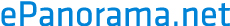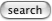# Generating DTMF tones using soundcard

## What are DTMF tones ?

DTMF tones are the tones used in telephones for tone dialling. The DTMF tones are sums of two sine wave tones at following frequencies:

```                 1209 Hz 1336 Hz 1477 Hz 1633 Hz

ABC     DEF
697 Hz          1       2       3       A

GHI     JKL     MNO
770 Hz          4       5       6       B

PRS     TUV     WXY
852 Hz          7       8       9       C

oper
941 Hz          *       0       #       D

```

## Methods for generating DTMF tones

• Use special IC for generating DTMF tones. Modems and telephones use this method.
• Generate DTMF tones using soundcard FM syntetizer chip
• Load sinewave sample to wavetable soundcard memeory. Play that sample using two instrument channels at different frequencies.
• Sample all DTMF tone combinations heeded and playback those samples as needed. 8 kHz at 8 bit resolution is enough for that.
• Genrate the sample data which is played back using software.
My test is concentrating on the last method, because it is the most generic way to do the DTMF generation. You can use this method with every sound card which can play back samples and it is as well suitable for DSP implementations also.

## How to generate DTMF tone samples

Generating sine wave samples is easy using the following formula:
sample=sin(n*2*pi*f/samplerate)
where

• n is the sample number (starting from 0)
• f is the frequency you wan to generate
• samplerate is the rate you are playing the samples through your soundcard DAC

Generating DTMF tones using this method is quite easy by just summing two of theose sine waves. For DTMF tones 8 kHz sample rate at 8 bit resolution is well enough, but there is no problems using 16 bit resolution or higher samplerates (you just have to generate more data for that).

Calculating samples for 8 kHz sample rate at 8 bit (unsigned) data, use the following function:
sample(n) = 128 + 63*sin(n*2*pi*f1/8000) + 63*sin(n*2*pi*f2/8000)
Where f1 and f2 are the frequencies of the sine waves in DTMF tone.

## How to speed up calculations

Calculating sin function is quite time consuming. If your application has limited amount of processing power available, you might want to optimize the routine in some way. The optimization in sin calculation can be easily done by calculating a sime table and then reading the sine values from that table instead of calculating actual sin function every time. Aother option is to use an algorithm to efficiently perform a series of sine and/or cosine calculations of an angle which is repeatedly increasing (or decreasing) by a fixed amount.

Other methods are to avoid doing unnecessary multiplications for every sample. You can calculate 2*pi*f1 and 2*pi*f2 once in the beginning of the DTMF tone calulation and use that stored value threreafter instead of doing it all over for every sample.

Tomi Engdahl <[email protected]>